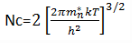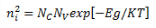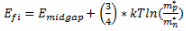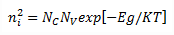Courses

# Test: Electrons And Holes In Semiconductor

## 10 Questions MCQ Test Electronic Devices | Test: Electrons And Holes In Semiconductor

Description
This mock test of Test: Electrons And Holes In Semiconductor for Electrical Engineering (EE) helps you for every Electrical Engineering (EE) entrance exam. This contains 10 Multiple Choice Questions for Electrical Engineering (EE) Test: Electrons And Holes In Semiconductor (mcq) to study with solutions a complete question bank. The solved questions answers in this Test: Electrons And Holes In Semiconductor quiz give you a good mix of easy questions and tough questions. Electrical Engineering (EE) students definitely take this Test: Electrons And Holes In Semiconductor exercise for a better result in the exam. You can find other Test: Electrons And Holes In Semiconductor extra questions, long questions & short questions for Electrical Engineering (EE) on EduRev as well by searching above.
QUESTION: 1

### Which of the following expressions represents the correct distribution of the electrons in the conduction band? (gc(E)=density of quantum states, fF(E)=Fermi dirac probability

Solution:

The distribution of the electrons in the conduction band is given by the product of the density into Fermi-dirac distribution.

QUESTION: 2

### What is the value of the effective density of states function in the conduction band at 300k?

Solution:Substituting the values of mn=m0 ,h=6.626*10-34J/s ,k=1.38*10-23 and T=300K, we get
Nc=2.5*1019 cm-3.

QUESTION: 3

### In a semiconductor which of the following carries can contribute to the current?

Solution:

In a semiconductor, two types of charges are there by which the flow of the current takes place. So, both the holes and electrons take part in the flow of the current.

QUESTION: 4

Which of the following expressions represent the Fermi probability function?

Solution:

It is the correct formula for the Fermi probability function.

QUESTION: 5

Electrons from valence band rises to conduction band when the temperature is greater than 0 k. Is it True or False?

Solution:

As the temperature rises above 0 k, the electrons gain energy and rises to the conduction band from the valence band.

QUESTION: 6

What is the intrinsic electrons concentration at T=300K in Silicon?

Solution:

Using the formula,We get, ni=1.5*1010cm-3.

QUESTION: 7

The intrinsic Fermi level of a semiconductor depends on which of the following things?

Solution:From the above formula, Efidepends on all of the options given.

QUESTION: 8

What is the difference between the practical value and theoretical value of ni?

Solution:

This is practically proved.

QUESTION: 9

The thermal equilibrium concentration of the electrons in the conduction band and the holes in the valence band depends upon?

Solution:

The electrons and holes depends upon the effective density of the states and the Fermi energy level given by the formula,QUESTION: 10

In which of the following semiconductor, the concentration of the holes and electrons is equal?

Solution:

In the intrinsic semiconductor, ni=pi that is the number of the electrons is equal to the number of the holes. Whereas in the extrinsic conductor ni is not equal to pi.